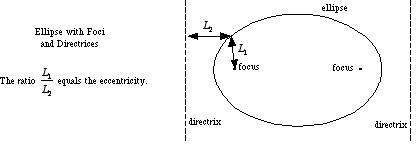index: click on a letter A B C D E F G H I J K L M N O P Q R S T U V W X Y Z A to Z index index: subject areas numbers & symbols sets, logic, proofs geometry algebra trigonometry advanced algebra & pre-calculus calculus advanced topics probability & statistics real world applications multimedia entrieswww.mathwords.com about mathwords website feedback

 Directrices of an Ellipse Two parallel lines on the outside of an ellipse perpendicular to the major axis. Directrices can be used to define an ellipse. Formally, an ellipse is the locus of points such that the ratio of the distance to the nearer focus to the distance to the nearer directrix equals a constant that is less than one. This constant is the eccentricity.See also